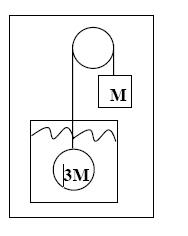# Density of a Submerged Ball

## Homework Statement

A block of mass M is attached to a light string. The string passes over a massless, frictionless pulley and is attached to a solid uniform ball of mass 3M. The ball is at rest and completely submerged in a fluid as shown. What is the ratio of the density of the fluid to the ball?Fb = (rho)Vg
Fg = mg

## The Attempt at a Solution

The forces acting upward must balance the forces acting downward on the ball. Thus,

Fb + Tension = Fg

Then,

(rho)Vg + T = 3Mg

We know that the tension in the string is equal to M because it is keeping the block at rest. Thus,

(rho)Vg + Mg = 3Mg
(rho)Vg = 2Mg

Divide both sides by Vg and we obtain:

(rho(fluid)) = 2(rho(ball))

This is not the correct answer. Can someone explain what I'm doing wrong?

LowlyPion
Homework Helper
What is the buoyant force on the ball?

It has 3*M*g acting down - its weight. It is in equilibrium so the buoyant force must be 2*M*g

The weight of displaced water then is density water times Volume = 2*M*g

But in that same volume is 3*M*g

Consider then the ratio of the weights of the ball, and the displaced water. Won't that ratio be the ratio of the densities since the volume is he same?

What is the buoyant force on the ball?

It has 3*M*g acting down - its weight. It is in equilibrium so the buoyant force must be 2*M*g

The weight of displaced water then is density water times Volume = 2*M*g

But in that same volume is 3*M*g

Consider then the ratio of the weights of the ball, and the displaced water. Won't that ratio be the ratio of the densities since the volume is he same?

Ahh, you're a lifesaver. Thank you!